# Plasma Angular Equations of Motion

The full plasma equation of motion, (2.331), can be written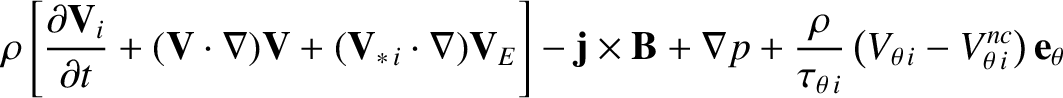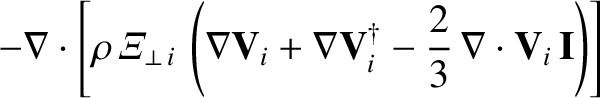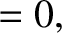(3.156)

where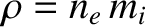is the plasma mass density,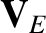the E-cross-B velocity [see Equation (2.138)],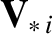the ion diamagnetic velocity [see Equation (2.139)],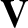the MHD velocity [see Equation (2.321)],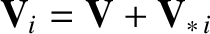the ion fluid velocity,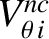the neoclassical poloidal velocity [see Equation (2.330)],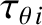the poloidal flow-damping time [see Equation (2.332)], and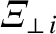the perpendicular ion momentum diffusivity. In writing the previous equation, we have assumed that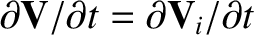because changes in the ion fluid rotation induced by tearing modes are mostly due to changes in the MHD velocity, rather than changes in the ion diamagnetic velocity (which is fixed by the ion pressure profile).

Now, according to the analysis of Section 2.25, the dominant terms in the previous equation are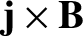and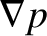. However, if we either take the poloidal flux-surface integral,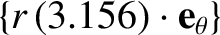, or the toroidal flux-surface integral,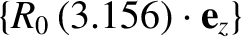, of this equation then theterm is completely annihilated (because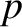is a single-valued function of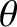and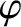), and theterm is largely annihilated. In fact, as was demonstrated in the previous section, the residualterm is quadratic in perturbed quantities, and localized in the vicinity of the rational surface. In these circumstance, it makes sense to include contributions from the other smaller terms in Equation (3.156). We shall calculate these contributions using the lowest-order (i.e., neglecting the contribution of the tearing perturbation) ion flow,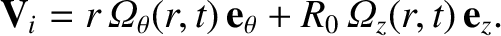(3.157)

Here,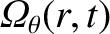and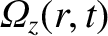are the ion poloidal and toroidal angular velocity profiles, respectively.

Taking, where the flux-surface integration operator is defined in Equation (3.131), we find that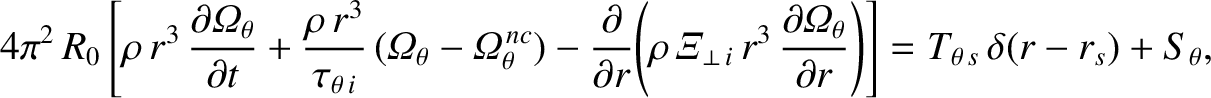(3.158)

where use has been made of Equations (3.133) and (3.157). Note that the advective inertia terms in Equation (3.156) make no contribution to the previous equation because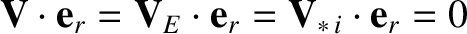. Here,. Moreover,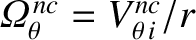. Here, we have added a source term,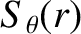, to the previous equation in order to account for the equilibrium flow. Let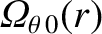be the unperturbed (by the tearing mode) ion poloidal angular velocity profile. It follows that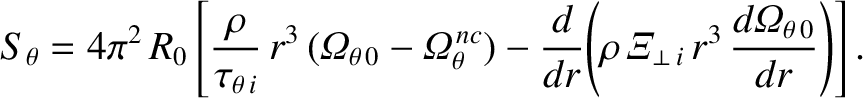(3.159)

Hence, writing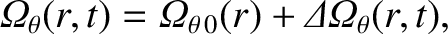(3.160)

where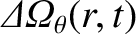is the modification to the ion poloidal angular velocity profile induced by the tearing mode, Equation (3.158) yields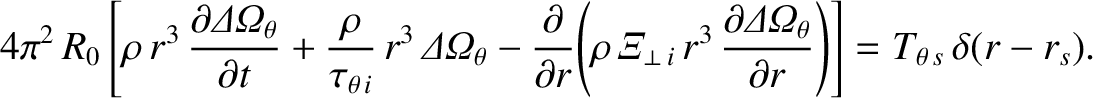(3.161)

Taking, we find that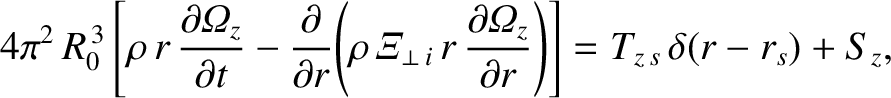(3.162)

where use has been made of Equations (3.134) and (3.157). We have again added a source term,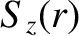, to the previous equation in order to account for the equilibrium flow. Let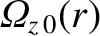be the unperturbed (by the tearing mode) ion toroidal angular velocity profile. It follows that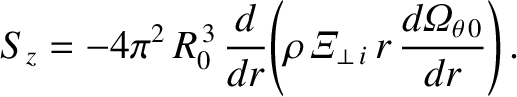(3.163)

Hence, writing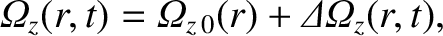(3.164)

where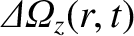is the modification to the ion toroidal angular velocity profile induced by the tearing mode, Equation (3.162) yields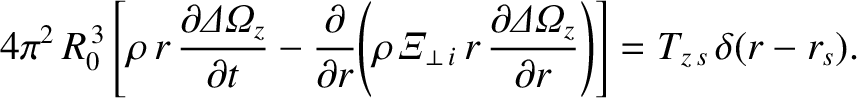(3.165)

Equations (3.161) and (3.165) are subject to the boundary conditions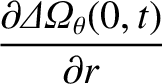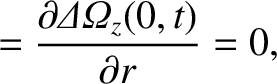(3.166)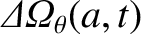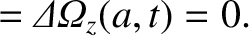(3.167)

The boundary conditions (3.166) merely ensure that the ion angular velocities remain finite at the magnetic axis. On the other hand, the boundary conditions (3.167) are a consequence of the action of charge exchange with electrically neutral particles emitted isotropically from the wall in the edge regions of the plasma [2,4,8]. Charge exchange with neutrals gives rise to dominant damping torques acting at the edge of the plasma that relax the edge ion angular velocities toward particular values. Moreover, the electromagnetic torques that develop at the rational surface are not large enough, compared with the charge-exchange torques, to significantly modify the edge ion angular velocities .